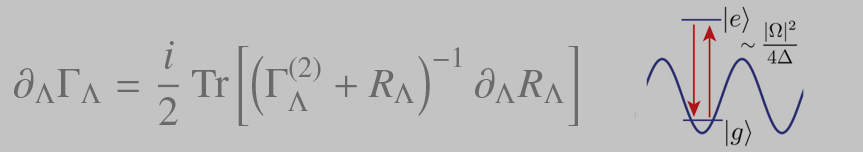## Lecture

Master

14756 | Quantum Error Correction
Monday 10:00 to 11.30, Room 0.02 New Building: Exercises
Wednesday 12.00 bis 13.30, Room 0.02 New Building: Lecture
First course: Wednesday October 10

Tutor:
Dr. Chae-Yeun Park (cypark [at] thp.uni-koeln [dot] de)

This course provides a modern introduction to the subject of quantum error correction, with an emphasis on topological error correction and its connections to many body theory. The course should bring student up to date with the latest developments in the field. Masters projects relating to course material will likely be available towards the end of the semester.

Lecture Notes updated on 03.02.19

• Exercise Sheet 1: Classical Linear codes [ PDF, Solutions]
• Exercise Sheet 2: Quantum Theory of a single qubit [ PDF, Solutions]
• Exercise Sheet 3: The Shor code [ PDF, Solutions]
• Exercise Sheet 4: Stabilizer codes [ PDF, Solutions]
• Exercise Sheet 5: The Toric Code [ PDF], Solutions]
• Exercise Sheet 6: Statistical mechanics of the toric code [ PDF, Solutions]
• Exercise Sheet 7: Bounds on [n,k,d] [ PDF, Solutions]
• Exercise Sheet 8: Davies Master equation [ PDF](due on Dec. 17)(Breuer book chapter PDF)
• Exercise Sheet 9: Fault-tolerance [ PDF](due on Jan. 28)

• Important update: Exercises do No exercises on the Color code lecture.

Topics to be covered:
- Basics of classical error correction; repetition code; code distance and rates; parity check operators.
- Basics of quantum error correction; stabiliser codes; Shor code; quantum error correction conditions; concatenation codes; sources of error; threshold theorems.
- Topological codes; the surface code; the color code; topological order; subsystem codes; gauge codes; mapping to statistical mechanics models
- Decoding; minimum weight perfect matching; other decoders
- Fault tolerance and operations; clifford gates; Easton-Knill theorem; magic state distillation; braiding and twists; gauge fixing.
- Self correction and finite temperature topological order; no-go theorems; partial self correction; fracton codes
- Advanced topics: quantum LDPC codes; approximate quantum error correction; tensor network codes

Literature:
M. Nielsen and Isaac Chuang, "Quantum Computation and Information", Cambridge University Press – Basic introduction to quantum information science.
D. Lidar and T. Brunn, "Quantum Error Correction", Cambridge University Press – Some good chapters, especially the one by Bombin.

Previous Courses

-->